## Who discovered the math of the digital polar discriminator?Started by 3 years ago25 replieslatest reply 3 years ago417 views

Good morning, everyone!

I've been researching the history of digital demodulation, and Rick Lyons passed this thesis paper written by James Shima on to me. In it, Mr. Shima discusses the digital polar discriminator, and how it can be implemented by calculating the product of a complex sample and the complex conjugate of the previous sample. This is shown in Figure 4.2 of his paper. I'm trying to nail down who discovered the math behind this. I've read through two, different linear algebra texts, but none of the parts on complex sampling discuss it. I've also checked through IEEE holdings (though I can only see abstracts, and not the entire text), all to no avail. It's quite possible Shima discovered this himself, as I'm having difficulty finding any prior art. His thesis does not make this clear. His references are either digital chip spec sheets, none of which discuss the discriminator, or standard college engineering texts. The closest I've come is a Hewlett-Packard announcement in 1992 talking about their new vector signal analyzer. I worked with that box when it came out, and it had digital demod capabilities. Mind you, I don't know if it used the polar discriminator or not.

Thank you for any assistance you can provide!

[ - ]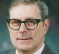I don't know if it is as simple as this. If a waveform has the component

x(nT) = Aexp(j n omega T) then the product of the sample with its predecessor's complex conjugate is

AA*exp(j n omega T)A*exp(-j (n-1) omega T)  = |A|^2 exp(j omega T )

If there are numerous components with A_i and omega_i

the  terms |A_i|^2 exp(j omega_i T) will all be added together and the    cross products will have a non-zero frequency (omega_i - omega_j) so they can be averaged out.

[ - ]In this case, it is simple. We're looking at this from the point of a FM signal. A digital version represented by complex samples will only have an instantaneous phase due to the modulation at one point. And the amplitude doesn't matter.

[ - ]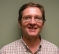I don't know where that term came from.  Taking the derivative of the phase to get instantaneous frequency dates back a long, long time.

This and other means of FSK demodulation are discussed in detail in:

Digital Signal Processing in Communication Systems

Marvin E. Frerking

I see recent patents on stuff that I can point to in the Frerking book.

[ - ]Thanks for the reply. Does Frerking explicitly mention calculating phase difference between consecutive samples by multiplying one sample with the conjugate of the previous one?

[ - ]Hi Gsparky2004. I think napierm is correct. I searched my copy of Frerking's book and the Section titled "Digital Differentiation" goes from page 253 to 257. I see that in July 2004 I wrote the following note in ink on page 255, "This is Shima's polar discriminator".

Frerking's book was copyrighted in 1994. Jim Shima's thesis is dated 1995.

[ - ]Thanks, Rick!

[ - ]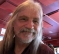maybe you should just credit Jim Shima for the math that is in his own thesis.

but it's not uncommonly known that the difference in angles or arguments is

$$\arg \{x[n]\} - \arg \{x[n-1]\} = \arg \left\{ \frac{x[n]}{x[n-1]} \right\} = \arg \{ x[n] x[n-1]^* \}$$

we use this fact, not only for instantaneous frequency, but we use it to naturally unwrap phase and get group delay in the frequency response of a filter.

i still haven't figured out how to do math on this site.

[ - ]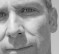Let me try.. $$\arg \{x[n]\} - \arg \{x[n-1]\} = \arg \left\{ \frac{x[n]}{x[n-1]} \right\} = \arg \{ x[n] x[n-1]^* \}$$

Check out the instructions here:

[ - ]Another try (inline): $$\arg \{x[n]\} - \arg \{x[n-1]\} = \arg \left\{ \frac{x[n]}{x[n-1]} \right\} = \arg \{ x[n] x[n-1]^* \}$$

[ - ]Stephane, if you can see the edit history of this post, i tried it all ways including with the double $. it never works when i do it. [ - ] Reply by May 2, 2020Hi Robert, what I would suggest is to look at the 'source' (html) of your post and remove from the equation anything that has been added by the editor. I don't know why it does that for you but your equation is polluted by tags that have been added by the editor used by this forum. To edit the source, first make sure to have your cursor active in the editor (by clicking anywhere inside) and just click on the <> button on the menu bar. Maybe one trick the next time you try to add an equation to your post would be to write it in Notepad and the copy/paste it in the editor. This seems to work well for me. Do not hesitate to reach out to me the next time you have issues with posting an equation, I would really like this feature to work for you. Thanks Robert. [ - ] Reply by May 2, 2020Hmmm. I tried your mathjax on the Mathjax.org demo page, and it rendered perfectly. I don't think the problem is your math. Rather than try to make the math work here, I'm just going to try for an image (NOTE: This was your math copied into the Mathjax demo page):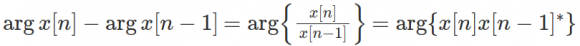The first part is straightforward. Calculate the phase of each complex sample, then subtract the previous one from the current one. The other two are what Shima stated in his thesis. Here's the rub. The first equation is not the same as the last two. The "argument" function returns a value between -pi and +pi. If the two samples straddle the -pi / +pi line, then that equation will return a value that is close to either -2*pi or +2*pi. The last two equations do not suffer this problem. That's what makes them so elegant. And the last two ONLY work if you're working with complex samples using Cartesian coordinates; they don't work if you're trying to it with the samples in polar form. Otherwise, you wind up with the -pi / +pi straddling problem again. With the samples in Cartesian form, you don't have this problem, and a digital FM discriminator becomes quite simple (overlooking the problem of having to calculate the arctangent function). The reason I'm asking this question goes back to your first comment. If Shima DID discover this (and, again, he does not make that clear in his paper), then I'll sing his praises to the sky. This is a brilliant piece of engineering, and I'd like to give proper credit to whomever discovered it. [ - ] Reply by May 2, 2020i still haven't figured out how to do math on this site. It turns our your code is fine; the only change that @stephaneb made below is he used a double dollar sign at the beginning and end, rather than a single dollar sign, AND (I think) he set the paragraph type to "inline code". I'm going to try it here: $$\arg \{x[n]\} - \arg \{x[n-1]\} = \arg \left\{ \frac{x[n]}{x[n-1]} \right\} = \arg \{ x[n] x[n-1]^* \}$$ This is interesting because, part of the reason I started down this rabbit hole is that Gnu Radio's documentation for their quadrature demod (which uses the polar discriminator method) says one thing in the text, but the TeX command (which my computer does not render properly) just below it says something else. [ - ] Reply by May 2, 2020still doesn't work for me. and i had orginally tried it with the double$.

[ - ]Maybe I'm missing something, but what is not trivial about this?   In his thesis he shows the simple relationship of the discriminator in Eq. 4.7, which I can't describe as anything beyond trivial.  You want the difference in the phase angles, and the product of two phasors multiplies the amplitudes and adds the angles...that's basic complex arithmetic, there's nothing new or exciting about that.   If the amplitude is not of interest, like in FM, or PSK, or anything with a fixed-modulus or inconsequential amplitude, then the amplitude doesn't matter at all, you can run it through a limiter (like in FM) and make it inconsequential.

So finding the difference in phase angle, to determine either phase modulation or frequency, means conjugating one of the arguments.

This has been done forever to demodulate differential PSK, and in modems for frequency or phase discrimination for synchronization.  We were doing it in LUTs, where the I and Q components of the current and previous symbols were used to compute the frequency and/or phase difference at least by the early 90s and probably before. Four bits of each I and Q component only required a 64k ROM to implement it.  I don't remember anybody thinking it was especially innovative at the time, it was just the easy and obvious way to do it.

You may have difficulty finding references for it because it isn't especially difficult or novel.   Unless I'm missing something?   Is there more to it?

Puzzled trying to sort out what the big deal is...

[ - ]I also see the complex conjugate multiplication method used to find the course frequency offset for GMSK/O-QPSK control loops.

It would be hard to find the original author of many of these, so much is never published.  I see stuff patented now that I saw already baked into hardware in the 90's.  As others have shown the math comes from the analog world.  So the engineer does some homework and figures out how to apply it on the digital side.  It's not surprising that the same solutions keep coming up.

That being said there are several nice tricks on that web site:

www.dspdude.com

[ - ]You want the difference in the phase angles, and the product of two phasors multiplies the amplitudes and adds the angles...that's basic complex arithmetic, there's nothing new or exciting about that.

True enough. Except in HOW you do it makes all the difference in the world. The first way is brute force. Treat each complex sample in polar form (magnitude, phase), subtract one phase from the phase that comes after it, and you have the phase difference. Except you're operating in the digital domain. If the two phases are straddling the $$- \pi / + \pi$$ line, then the absolute difference will be greater than $$\pi$$. That violates Nyquist. So, how do you fix that? You could add an if/then statement after the calculation to correct it. Or you could convert the sample from polar back to Cartesian form, then BACK to polar form.

Or you could leave the samples in Cartesian form BEFORE you calculate the phase angle. Calculate the product of one with the conjugate of the previous one. That does the phase subtraction BEFORE you've actually calculated the phase. That beautifully and elegantly deals with the $$\pi$$ line straddling problem. It's also quite efficient from a system resources (four multiplies and two adds per complex multiply).

I realize (as napierm has stated) that many of these techniques were developed in the analog world, but this particular technique solves a problem unique to the digital world. And I simply wanted to give proper credit. I'd only seen it in Shima's paper. I'd asked Rick Lyons (by e-mail), and he suggested I ask this forum. Napeierm pointed out that it was in Frerking's textbook. So Shima was not the one who discovered it. Don't worry. I'm not going to go down this rabbit hole any further.

[ - ]Remember that in the vast majority of implementations the quantities will already be cartesian, specifically I and Q components of a signal.  So doing the operation in cartesian coordinates is generally the default.   As I mentioned, doing those kinds of things in a ROM, which was pretty common in the 80s and 90s, meant only the solutions were stored in the memory, so the software that generated the ROM content could do whatever normalization or unwrapping or whatever that was needed.

Later when multipliers became cheap in silicon or FPGAs or DSPs, then it became more common to just do the complex arithmetic in the implementation.  None of that changed the basic concept, but usually implementers were dealing with cartesian complex quantities.

[ - ]Analog FM precedes DSP by years, and so does the math.  I cannot find a reference, but I dubbed this process a Homodyne discriminator in my book.  A Google search for homodyne discriminator only shows derivatives from my book.  The math is pretty basic.

Here's the math, where the samples are one unit apart: (forgive the limits of pure text):

R*e^(jwt)*R*e^(-jwt[-1])= R^2 * e^(jw(t - t[-1])) = R^2*e^(jw)

[ - ]Let me see if I can parse out your equation:

$$R \exp \{ j \omega t \} \times R \exp \{ j \omega t [-1] \} = R^2 \exp \{ j \omega \left( t - t[-1] \right) \} = R^2 \exp \{ j \omega \}$$

Do I have that correct?

[ - ]looks good

[ - ]Cool! Thank you!

The issue is that, when you do this in the digital domain, the polar form of the math will give you the wrong value if the two samples being used straddle the $$- \pi$$ / $$+ \pi$$ boundary. If you use the Cartesian coordinates, and either divide the previous sample from the current one (or multiply the conjugate of the previous sample with the current one, which is the same thing), the phase will always wind up between $$- \pi$$ / $$+ \pi$$. And, when used in a frequency discriminator, you wind up with a value that can be scaled to properly represent the baseband information.

So, the question is this: Someone (and it appears to have been Shima), figured out that using the Cartesian values in the math, rather than the polar form, will work ideally for a digital FM discriminator. I'm just trying to confirm who that person (or group) was.

Thanks, again!

[ - ]Does this work as an equivalent?

$$R e^{j\omega t}*Re^{-jwt[-1]}= R^2 * e^{jw(t - t[-1])} = R^2*e^{j\omega}$$

I don't see how $$t - t$$ becomes 1 in the last step.

[ - ]